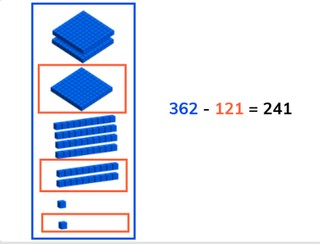Subtraction to 1,000 without crossing tens or hundreds

# Subtraction to 1,000 without crossing tens or hundreds

Learn to subtract to 1,000 without crossing tens or hundreds.

No account needed.8,000 schools use Gynzy92,000 teachers use Gynzy1,600,000 students use Gynzy

## General

Students learn to subtract numbers to 1,000 without crossing tens or hundreds.

## Standards

CCSS.Math.Content.2.NBT.B.7

## Learning objective

Students will be able to subtract to 1,000 without crossing tens or hundreds.

## Introduction

Ask students to determine how many bananas are left. Have students explain their strategy out loud as they solve the problems.

## Development

Explain that you can subtract by counting. You first count how many there are in total, this is the first number of the subtraction problem (the minuend). Then you count how many are taken away, which is the second number of the subtraction problem (the subtrahend). When you take the second from the first you are left with the difference. Show this on the interactive whiteboard using the blocks, and discuss the different problems. This includes problems in which the second number (the subtrahend) has hundreds, tens, and ones. Check that students are able to solve a few problems on their own or in pairs with the given problems with blocks.Ask them to say the problem they are solving out loud, and to say the steps they are taking out loud. Next explain that you can solve these subtraction problems in different ways. Show that you can use a number line to subtract. You can also split the subtrahend and take away the hundreds, tens, and ones in steps. You can also choose to take away the subtrahend in one step. Emphasize to students that they should first carefully look at the problem and then decide which method to use, because all of them work, but some are easier to use on different math problems. Ask students to solve a few problems individually or in pairs, explaining their strategy. Finally walk through the steps of a story problem with the class. Explain that you first determine what kind of math problem it is, then you determine which numbers are important for the problem. You say or write the problem and finally solve the problem. Do one story problem as a class and then ask students to solve another individually or in pairs.

Check that students are able to subtract numbers to 1,000 without crossing tens or hundreds by asking the following questions:
- What do you look at when you have to solve a story problem?
- How do you solve 774 - 522?

## Guided Practice

Students are given subtraction problems with visual support, as abstract problems, and story problems. Have students explain the steps they are taking to solve the problems out loud, or to a partner.

## Closing

Discuss with students that it is important to be able to subtract numbers to 1,000 without crossing tens or hundreds so that you can determine the difference of larger numbers. Ask students to solve the subtraction problems on the board. Ask students to explain their strategy. How did they solve the problem? Close by having students work in pairs. At the top of the stairs is a number. As it falls down the stairs, it falls apart. How many are left when the number reaches the bottom?

## Teaching tips

Students who have difficulty with subtraction can use a number line as a visual support. Remind students that they can take different sized jumps on the number line.

## Instruction materials

Optional: MAB blocks or other manipulatives, number lines.

### The online teaching platform for interactive whiteboards and displays in schools

• Save time building lessons

• Manage the classroom more efficiently

• Increase student engagement